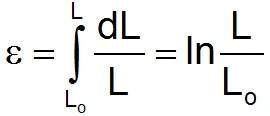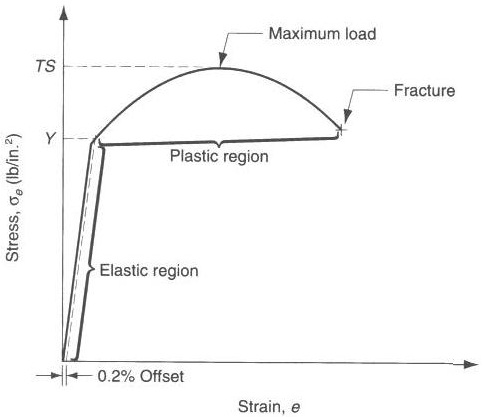Feb 26, 2016

Feb 26, 2016

Feb 26, 2016

# True Stress and Strain

Also see Engineering Stress and Strain

## True Stress

The true stress (ø) uses the instantaneous or actual area of the specimen at any given point, as opposed to the original area used in the engineering values.## True Strain

The true strain (e) is defined as the instantaneous elongation per unit length of the specimen.## Relation between True Stress and True Strain

The relationship between true stress and true strain i.e. the flow curve can be expressed using the power law:where K is called the strength coefficient and n the Strain Hardening exponent.

## Relation between True and Engineering Stress-Strain

For a given value of the load and elongation, the true stress is higher than the Engg. stress, while the true strain is smaller than the Engg. Strain. The relationship between the true and engineering values is given by the formula: